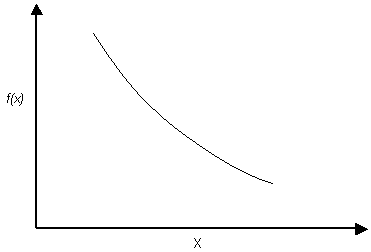The Rocscience International Conference 2021 Proceedings are now available. Read Now

# Exponential Distribution

The Exponential probability density function is often used to define random variables such as joint length or persistence.

Exponential probability density functionNOTE:

• The range of values must always be positive for an Exponential distribution, therefore it cannot be used for variables which include negative values.
• The mean is always equal to the standard deviation for an Exponential distribution. This is a property of the Exponential distribution, and cannot be altered by the user.
• The basic Exponential distribution varies from zero to infinity. Like the Normal distribution, the Exponential distribution can be truncated by entering the desired minimum and maximum values.